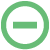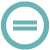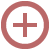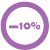(window.NREUM||(NREUM={})).init={privacy:{cookies_enabled:true},ajax:{deny_list:["bam.nr-data.net"]},distributed_tracing:{enabled:true}};(window.NREUM||(NREUM={})).loader_config={agentID:"1386068166",accountID:"3724901",trustKey:"3724901",xpid:"VwEFVV9TCRABVllTBwgHU10D",licenseKey:"NRJS-85661d126f8e771c55f",applicationID:"1281090592"};;(()=>{var e,t,r={9071:(e,t,r)=>{"use strict";r.d(t,{I:()=>n});var n=0,i=navigator.userAgent.match(/Firefox[\/\s](\d+\.\d+)/);i&&(n=+i)},8768:(e,t,r)=>{"use strict";r.d(t,{T:()=>n,p:()=>i});const n=/(iPad|iPhone|iPod)/g.test(navigator.userAgent),i=n&&Boolean("undefined"==typeof SharedWorker)},2919:(e,t,r)=>{"use strict";r.d(t,{P_:()=>g,Mt:()=>v,C5:()=>c,DL:()=>y,OP:()=>R,lF:()=>P,Yu:()=>E,Dg:()=>p,CX:()=>u,GE:()=>w,sU:()=>C});var n={};r.r(n),r.d(n,{agent:()=>A,match:()=>N,version:()=>x});var i=r(6797),o=r(4286);const a={beacon:i.ce.beacon,errorBeacon:i.ce.errorBeacon,licenseKey:void 0,applicationID:void 0,sa:void 0,queueTime:void 0,applicationTime:void 0,ttGuid:void 0,user:void 0,account:void 0,product:void 0,extra:void 0,jsAttributes:{},userAttributes:void 0,atts:void 0,transactionName:void 0,tNamePlain:void 0},s={};function c(e){if(!e)throw new Error("All info objects require an agent identifier!");if(!s[e])throw new Error("Info for ".concat(e," was never set"));return s[e]}function u(e,t){if(!e)throw new Error("All info objects require an agent identifier!");s[e]=new o.I(t,a),(0,i.Qy)(e,s[e],"info")}var d=r(2797),f=r(2374);const l={allow_bfcache:!0,privacy:{cookies_enabled:!0},ajax:{deny_list:void 0,enabled:!0,harvestTimeSeconds:10},distributed_tracing:{enabled:void 0,exclude_newrelic_header:void 0,cors_use_newrelic_header:void 0,cors_use_tracecontext_headers:void 0,allowed_origins:void 0},session:{domain:void 0,expiresMs:d.oD,inactiveMs:d.Hb},ssl:void 0,obfuscate:void 0,jserrors:{enabled:!0,harvestTimeSeconds:10},metrics:{enabled:!0},page_action:{enabled:!0,harvestTimeSeconds:30},page_view_event:{enabled:!0},page_view_timing:{enabled:!0,harvestTimeSeconds:30,long_task:!1},session_trace:{enabled:!0,harvestTimeSeconds:10},spa:{enabled:!0,harvestTimeSeconds:10}},h={};function g(e){if(!e)throw new Error("All configuration objects require an agent identifier!");if(!h[e])throw new Error("Configuration for ".concat(e," was never set"));return h[e]}function p(e,t){if(!e)throw new Error("All configuration objects require an agent identifier!");h[e]=new o.I(t,l),(0,i.Qy)(e,h[e],"config")}function v(e,t){if(!e)throw new Error("All configuration objects require an agent identifier!");var r=g(e);if(r){for(var n=t.split("."),i=0;i<n.length-1;i++)if("object"!=typeof(r=r[n[i]]))return;r=r[n[n.length-1]]}return r}const m={accountID:void 0,trustKey:void 0,agentID:void 0,licenseKey:void 0,applicationID:void 0,xpid:void 0},b={};function y(e){if(!e)throw new Error("All loader-config objects require an agent identifier!");if(!b[e])throw new Error("LoaderConfig for ".concat(e," was never set"));return b[e]}function w(e,t){if(!e)throw new Error("All loader-config objects require an agent identifier!");b[e]=new o.I(t,m),(0,i.Qy)(e,b[e],"loader_config")}const E=(0,i.mF)().o;var A=null,x=null;const T=/Version\/(\S+)\s+Safari/;if(navigator.userAgent){var _=navigator.userAgent,D=_.match(T);D&&-1===_.indexOf("Chrome")&&-1===_.indexOf("Chromium")&&(A="Safari",x=D)}function N(e,t){if(!A)return!1;if(e!==A)return!1;if(!t)return!0;if(!x)return!1;for(var r=x.split("."),n=t.split("."),i=0;i<n.length;i++)if(n[i]!==r[i])return!1;return!0}var S=r(1651);const O={buildEnv:S.Re,bytesSent:{},queryBytesSent:{},customTransaction:void 0,disabled:!1,distMethod:S.gF,isolatedBacklog:!1,loaderType:void 0,maxBytes:3e4,offset:Math.floor(f._A?.performance?.timeOrigin||f._A?.performance?.timing?.navigationStart||Date.now()),onerror:void 0,origin:""+f._A.location,ptid:void 0,releaseIds:{},session:void 0,xhrWrappable:"function"==typeof f._A.XMLHttpRequest?.prototype?.addEventListener,userAgent:n,version:S.q4},j={};function R(e){if(!e)throw new Error("All runtime objects require an agent identifier!");if(!j[e])throw new Error("Runtime for ".concat(e," was never set"));return j[e]}function C(e,t){if(!e)throw new Error("All runtime objects require an agent identifier!");j[e]=new o.I(t,O),(0,i.Qy)(e,j[e],"runtime")}function P(e){return function(e){try{const t=c(e);return!!t.licenseKey&&!!t.errorBeacon&&!!t.applicationID}catch(e){return!1}}(e)}},4286:(e,t,r)=>{"use strict";r.d(t,{I:()=>o});var n=r(909),i=r(8610);class o{constructor(e,t){Object.assign(this,function(e,t){const r={};try{return e&&"object"==typeof e?t&&"object"==typeof t?(Object.assign(r,t),Object.entries(e).forEach((e=>{let[o,a]=e;if(!Object.keys(t).includes(o))return;const s=(0,n.q)(o);s.length&&a&&"object"==typeof a&&s.forEach((e=>{e in a&&((0,i.Z)('"'.concat(e,'" is a protected attribute and can not be changed in feature ').concat(o,". It will have no effect.")),delete a[e])})),r[o]=a})),r):(0,i.Z)("Setting a Configurable requires a model to set its initial properties"):(0,i.Z)("New setting a Configurable requires an object as input")}catch(e){(0,i.Z)("An error occured while setting a Configurable",e)}}(e,t))}}},1651:(e,t,r)=>{"use strict";r.d(t,{Re:()=>i,gF:()=>o,q4:()=>n});const n="1.232.0",i="PROD",o="CDN"},9557:(e,t,r)=>{"use strict";r.d(t,{w:()=>o});var n=r(8610);const i={agentIdentifier:"",ee:void 0};class o{constructor(e){try{if("object"!=typeof e)return(0,n.Z)("shared context requires an object as input");this.sharedContext={},Object.assign(this.sharedContext,i),Object.entries(e).forEach((e=>{let[t,r]=e;Object.keys(i).includes(t)&&(this.sharedContext[t]=r)}))}catch(e){(0,n.Z)("An error occured while setting SharedContext",e)}}}},4329:(e,t,r)=>{"use strict";r.d(t,{L:()=>d,R:()=>c});var n=r(3752),i=r(7022),o=r(4045),a=r(2325);const s={};function c(e,t){const r={staged:!1,priority:a.p[t]||0};u(e),s[e].get(t)||s[e].set(t,r)}function u(e){e&&(s[e]||(s[e]=new Map))}function d(){let e=arguments.length>0&&void 0!==arguments?arguments:"",t=arguments.length>1&&void 0!==arguments?arguments:"feature";if(u(e),!e||!s[e].get(t))return a(t);s[e].get(t).staged=!0;const r=Array.from(s[e]);function a(t){const r=e?n.ee.get(e):n.ee,a=o.X.handlers;if(r.backlog&&a){var s=r.backlog[t],c=a[t];if(c){for(var u=0;s&&u<s.length;++u)f(s[u],c);(0,i.D)(c,(function(e,t){(0,i.D)(t,(function(t,r){r.on(e,r)}))}))}delete a[t],r.backlog[t]=null,r.emit("drain-"+t,[])}}r.every((e=>{let[t,r]=e;return r.staged}))&&(r.sort(((e,t)=>e.priority-t.priority)),r.forEach((e=>{let[t]=e;a(t)})))}function f(e,t){var r=e;(0,i.D)(t[r],(function(t,r){var n=e;if(r===n){var i=r,o=e,a=e;i.apply(o,a)}}))}},3752:(e,t,r)=>{"use strict";r.d(t,{c:()=>f,ee:()=>u});var n=r(6797),i=r(3916),o=r(7022),a=r(2919),s="nr@context";let c=(0,n.fP)();var u;function d(){}function f(e){return(0,i.X)(e,s,l)}function l(){return new d}function h(){u.aborted=!0,u.backlog={}}c.ee?u=c.ee:(u=function e(t,r){var n={},c={},f={},g=!1;try{g=16===r.length&&(0,a.OP)(r).isolatedBacklog}catch(e){}var p={on:b,addEventListener:b,removeEventListener:y,emit:m,get:E,listeners:w,context:v,buffer:A,abort:h,aborted:!1,isBuffering:x,debugId:r,backlog:g?{}:t&&"object"==typeof t.backlog?t.backlog:{}};return p;function v(e){return e&&e instanceof d?e:e?(0,i.X)(e,s,l):l()}function m(e,r,n,i,o){if(!1!==o&&(o=!0),!u.aborted||i){t&&o&&t.emit(e,r,n);for(var a=v(n),s=w(e),d=s.length,f=0;f<d;f++)s[f].apply(a,r);var l=T()[c[e]];return l&&l.push([p,e,r,a]),a}}function b(e,t){n[e]=w(e).concat(t)}function y(e,t){var r=n[e];if(r)for(var i=0;i<r.length;i++)r[i]===t&&r.splice(i,1)}function w(e){return n[e]||[]}function E(t){return f[t]=f[t]||e(p,t)}function A(e,t){var r=T();p.aborted||(0,o.D)(e,(function(e,n){t=t||"feature",c[n]=t,t in r||(r[t]=[])}))}function x(e){return!!T()[c[e]]}function T(){return p.backlog}}(void 0,"globalEE"),c.ee=u)},9252:(e,t,r)=>{"use strict";r.d(t,{E:()=>n,p:()=>i});var n=r(3752).ee.get("handle");function i(e,t,r,i,o){o?(o.buffer([e],i),o.emit(e,t,r)):(n.buffer([e],i),n.emit(e,t,r))}},4045:(e,t,r)=>{"use strict";r.d(t,{X:()=>o});var n=r(9252);o.on=a;var i=o.handlers={};function o(e,t,r,o){a(o||n.E,i,e,t,r)}function a(e,t,r,i,o){o||(o="feature"),e||(e=n.E);var a=t[o]=t[o]||{};(a[r]=a[r]||[]).push([e,i])}},8544:(e,t,r)=>{"use strict";r.d(t,{bP:()=>s,iz:()=>c,m$:()=>a});var n=r(2374);let i=!1,o=!1;try{const e={get passive(){return i=!0,!1},get signal(){return o=!0,!1}};n._A.addEventListener("test",null,e),n._A.removeEventListener("test",null,e)}catch(e){}function a(e,t){return i||o?{capture:!!e,passive:i,signal:t}:!!e}function s(e,t){let r=arguments.length>2&&void 0!==arguments&&arguments,n=arguments.length>3?arguments:void 0;window.addEventListener(e,t,a(r,n))}function c(e,t){let r=arguments.length>2&&void 0!==arguments&&arguments,n=arguments.length>3?arguments:void 0;document.addEventListener(e,t,a(r,n))}},5526:(e,t,r)=>{"use strict";r.d(t,{Ht:()=>u,M:()=>c,Rl:()=>a,ky:()=>s});var n=r(2374);const i="xxxxxxxx-xxxx-4xxx-yxxx-xxxxxxxxxxxx";function o(e,t){return e?15&e[t]:16*Math.random()|0}function a(){const e=n._A?.crypto||n._A?.msCrypto;let t,r=0;return e&&e.getRandomValues&&(t=e.getRandomValues(new Uint8Array(31))),i.split("").map((e=>"x"===e?o(t,++r).toString(16):"y"===e?(3&o()|8).toString(16):e)).join("")}function s(e){const t=n._A?.crypto||n._A?.msCrypto;let r,i=0;t&&t.getRandomValues&&(r=t.getRandomValues(new Uint8Array(31)));const a=[];for(var s=0;s<e;s++)a.push(o(r,++i).toString(16));return a.join("")}function c(){return s(16)}function u(){return s(32)}},2797:(e,t,r)=>{"use strict";r.d(t,{Bq:()=>n,Hb:()=>o,oD:()=>i});const n="NRBA",i=144e5,o=18e5},2053:(e,t,r)=>{"use strict";function n(){return Math.round(performance.now())}r.d(t,{z:()=>n})},6368:(e,t,r)=>{"use strict";r.d(t,{e:()=>o});var n=r(2374),i={};function o(e){if(e in i)return i[e];if(0===(e||"").indexOf("data:"))return{protocol:"data"};let t;var r=n._A?.location,o={};if(n.il)t=document.createElement("a"),t.href=e;else try{t=new URL(e,r.href)}catch(e){return o}o.port=t.port;var a=t.href.split("://");!o.port&&a&&(o.port=a.split("/").split("@").pop().split(":")),o.port&&"0"!==o.port||(o.port="https"===a?"443":"80"),o.hostname=t.hostname||r.hostname,o.pathname=t.pathname,o.protocol=a,"/"!==o.pathname.charAt(0)&&(o.pathname="/"+o.pathname);var s=!t.protocol||":"===t.protocol||t.protocol===r.protocol,c=t.hostname===r.hostname&&t.port===r.port;return o.sameOrigin=s&&(!t.hostname||c),"/"===o.pathname&&(i[e]=o),o}},8610:(e,t,r)=>{"use strict";function n(e,t){"function"==typeof console.warn&&(console.warn("New Relic: ".concat(e)),t&&console.warn(t))}r.d(t,{Z:()=>n})},3916:(e,t,r)=>{"use strict";r.d(t,{X:()=>i});var n=Object.prototype.hasOwnProperty;function i(e,t,r){if(n.call(e,t))return e[t];var i=r();if(Object.defineProperty&&Object.keys)try{return Object.defineProperty(e,t,{value:i,writable:!0,enumerable:!1}),i}catch(e){}return e[t]=i,i}},2374:(e,t,r)=>{"use strict";r.d(t,{_A:()=>o,il:()=>n,v6:()=>i});const n=Boolean("undefined"!=typeof window&&window.document),i=Boolean("undefined"!=typeof WorkerGlobalScope&&self.navigator instanceof WorkerNavigator);let o=(()=>{if(n)return window;if(i){if("undefined"!=typeof globalThis&&globalThis instanceof WorkerGlobalScope)return globalThis;if(self instanceof WorkerGlobalScope)return self}throw new Error('New Relic browser agent shutting down due to error: Unable to locate global scope. This is possibly due to code redefining browser global variables like "self" and "window".')})()},7022:(e,t,r)=>{"use strict";r.d(t,{D:()=>n});const n=(e,t)=>Object.entries(e||{}).map((e=>{let[r,n]=e;return t(r,n)}))},2438:(e,t,r)=>{"use strict";r.d(t,{P:()=>o});var n=r(3752);const i=()=>{const e=new WeakSet;return(t,r)=>{if("object"==typeof r&&null!==r){if(e.has(r))return;e.add(r)}return r}};function o(e){try{return JSON.stringify(e,i())}catch(e){try{n.ee.emit("internal-error",[e])}catch(e){}}}},2650:(e,t,r)=>{"use strict";r.d(t,{K:()=>a,b:()=>o});var n=r(8544);function i(){return"undefined"==typeof document||"complete"===document.readyState}function o(e,t){if(i())return e();(0,n.bP)("load",e,t)}function a(e){if(i())return e();(0,n.iz)("DOMContentLoaded",e)}},6797:(e,t,r)=>{"use strict";r.d(t,{EZ:()=>u,Qy:()=>c,ce:()=>o,fP:()=>a,gG:()=>d,mF:()=>s});var n=r(2053),i=r(2374);const o={beacon:"bam.nr-data.net",errorBeacon:"bam.nr-data.net"};function a(){return i._A.NREUM||(i._A.NREUM={}),void 0===i._A.newrelic&&(i._A.newrelic=i._A.NREUM),i._A.NREUM}function s(){let e=a();return e.o||(e.o={ST:i._A.setTimeout,SI:i._A.setImmediate,CT:i._A.clearTimeout,XHR:i._A.XMLHttpRequest,REQ:i._A.Request,EV:i._A.Event,PR:i._A.Promise,MO:i._A.MutationObserver,FETCH:i._A.fetch}),e}function c(e,t,r){let i=a();const o=i.initializedAgents||{},s=o[e]||{};return Object.keys(s).length||(s.initializedAt={ms:(0,n.z)(),date:new Date}),i.initializedAgents={...o,[e]:{...s,[r]:t}},i}function u(e,t){a()[e]=t}function d(){return function(){let e=a();const t=e.info||{};e.info={beacon:o.beacon,errorBeacon:o.errorBeacon,...t}}(),function(){let e=a();const t=e.init||{};e.init={...t}}(),s(),function(){let e=a();const t=e.loader_config||{};e.loader_config={...t}}(),a()}},6998:(e,t,r)=>{"use strict";r.d(t,{N:()=>i});var n=r(8544);function i(e){let t=arguments.length>1&&void 0!==arguments&&arguments,r=arguments.length>2?arguments:void 0,i=arguments.length>3?arguments:void 0;return void(0,n.iz)("visibilitychange",(function(){if(t)return void("hidden"==document.visibilityState&&e());e(document.visibilityState)}),r,i)}},6408:(e,t,r)=>{"use strict";r.d(t,{W:()=>i});var n=r(2374);function i(){return"function"==typeof n._A?.PerformanceObserver}},7264:(e,t,r)=>{"use strict";r.d(t,{em:()=>y,u5:()=>O,QU:()=>C,_L:()=>H,Gm:()=>B,Lg:()=>F,gy:()=>G,BV:()=>K,Kf:()=>re});var n=r(3752),i=r(8683),o=r.n(i);const a="nr@original";var s=Object.prototype.hasOwnProperty,c=!1;function u(e,t){return e||(e=n.ee),r.inPlace=function(e,t,n,i,o){n||(n="");var a,s,c,u="-"===n.charAt(0);for(c=0;c<t.length;c++)l(a=e[s=t[c]])||(e[s]=r(a,u?s+n:n,i,s,o))},r.flag=a,r;function r(t,r,n,s,c){return l(t)?t:(r||(r=""),nrWrapper[a]=t,f(t,nrWrapper,e),nrWrapper);function nrWrapper(){var a,u,f,l;try{u=this,a=o()(arguments),f="function"==typeof n?n(a,u):n||{}}catch(t){d([t,"",[a,u,s],f],e)}i(r+"start",[a,u,s],f,c);try{return l=t.apply(u,a)}catch(e){throw i(r+"err",[a,u,e],f,c),e}finally{i(r+"end",[a,u,l],f,c)}}}function i(r,n,i,o){if(!c||t){var a=c;c=!0;try{e.emit(r,n,i,t,o)}catch(t){d([t,r,n,i],e)}c=a}}}function d(e,t){t||(t=n.ee);try{t.emit("internal-error",e)}catch(e){}}function f(e,t,r){if(Object.defineProperty&&Object.keys)try{return Object.keys(e).forEach((function(r){Object.defineProperty(t,r,{get:function(){return e[r]},set:function(t){return e[r]=t,t}})})),t}catch(e){d([e],r)}for(var n in e)s.call(e,n)&&(t[n]=e[n]);return t}function l(e){return!(e&&e instanceof Function&&e.apply&&!e[a])}var h=r(3916),g=r(2374);const p={},v=XMLHttpRequest,m="addEventListener",b="removeEventListener";function y(e){var t=function(e){return(e||n.ee).get("events")}(e);if(p[t.debugId]++)return t;p[t.debugId]=1;var r=u(t,!0);function i(e){r.inPlace(e,[m,b],"-",o)}function o(e,t){return e}return"getPrototypeOf"in Object&&(g.il&&w(document,i),w(g._A,i),w(v.prototype,i)),t.on(m+"-start",(function(e,t){var n=e;if(null!==n&&("function"==typeof n||"object"==typeof n)){var i=(0,h.X)(n,"nr@wrapped",(function(){var e={object:function(){if("function"!=typeof n.handleEvent)return;return n.handleEvent.apply(n,arguments)},function:n}[typeof n];return e?r(e,"fn-",null,e.name||"anonymous"):n}));this.wrapped=e=i}})),t.on(b+"-start",(function(e){e=this.wrapped||e})),t}function w(e,t){let r=e;for(;"object"==typeof r&&!Object.prototype.hasOwnProperty.call(r,m);)r=Object.getPrototypeOf(r);for(var n=arguments.length,i=new Array(n>2?n-2:0),o=2;o<n;o++)i[o-2]=arguments[o];r&&t(r,...i)}var E="fetch-",A=E+"body-",x=["arrayBuffer","blob","json","text","formData"],T=g._A.Request,_=g._A.Response,D="prototype",N="nr@context";const S={};function O(e){const t=function(e){return(e||n.ee).get("fetch")}(e);if(!(T&&_&&g._A.fetch))return t;if(S[t.debugId]++)return t;function r(e,r,n){var i=e[r];"function"==typeof i&&(e[r]=function(){var e,r=o()(arguments),a={};t.emit(n+"before-start",[r],a),a[N]&&a[N].dt&&(e=a[N].dt);var s=i.apply(this,r);return t.emit(n+"start",[r,e],s),s.then((function(e){return t.emit(n+"end",[null,e],s),e}),(function(e){throw t.emit(n+"end",[e],s),e}))})}return S[t.debugId]=1,x.forEach((e=>{r(T[D],e,A),r(_[D],e,A)})),r(g._A,"fetch",E),t.on(E+"end",(function(e,r){var n=this;if(r){var i=r.headers.get("content-length");null!==i&&(n.rxSize=i),t.emit(E+"done",[null,r],n)}else t.emit(E+"done",[e],n)})),t}const j={},R=["pushState","replaceState"];function C(e){const t=function(e){return(e||n.ee).get("history")}(e);return!g.il||j[t.debugId]++||(j[t.debugId]=1,u(t).inPlace(window.history,R,"-")),t}var P=r(8544);const I={},k=["appendChild","insertBefore","replaceChild"];function H(e){const t=function(e){return(e||n.ee).get("jsonp")}(e);if(!g.il||I[t.debugId])return t;I[t.debugId]=!0;var r=u(t),i=/[?&](?:callback|cb)=([^&#]+)/,o=/(.*)\.([^.]+)/,a=/^(\w+)(\.|$)(.*)$/;function s(e,t){var r=e.match(a),n=r,i=r;return i?s(i,t[n]):t[n]}return r.inPlace(Node.prototype,k,"dom-"),t.on("dom-start",(function(e){!function(e){if(!e||"string"!=typeof e.nodeName||"script"!==e.nodeName.toLowerCase())return;if("function"!=typeof e.addEventListener)return;var n=(a=e.src,c=a.match(i),c?c:null);var a,c;if(!n)return;var u=function(e){var t=e.match(o);if(t&&t.length>=3)return{key:t,parent:s(t,window)};return{key:e,parent:window}}(n);if("function"!=typeof u.parent[u.key])return;var d={};function f(){t.emit("jsonp-end",[],d),e.removeEventListener("load",f,(0,P.m$)(!1)),e.removeEventListener("error",l,(0,P.m$)(!1))}function l(){t.emit("jsonp-error",[],d),t.emit("jsonp-end",[],d),e.removeEventListener("load",f,(0,P.m$)(!1)),e.removeEventListener("error",l,(0,P.m$)(!1))}r.inPlace(u.parent,[u.key],"cb-",d),e.addEventListener("load",f,(0,P.m$)(!1)),e.addEventListener("error",l,(0,P.m$)(!1)),t.emit("new-jsonp",[e.src],d)}(e)})),t}var L=r(2919);const z={};function B(e){const t=function(e){return(e||n.ee).get("mutation")}(e);if(!g.il||z[t.debugId])return t;z[t.debugId]=!0;var r=u(t),i=L.Yu.MO;return i&&(window.MutationObserver=function(e){return this instanceof i?new i(r(e,"fn-")):i.apply(this,arguments)},MutationObserver.prototype=i.prototype),t}const M={};function F(e){const t=function(e){return(e||n.ee).get("promise")}(e);if(M[t.debugId])return t;M[t.debugId]=!0;var r=n.c,i=u(t),o=L.Yu.PR;return o&&function(){function e(r){var n=t.context(),a=i(r,"executor-",n,null,!1);const s=Reflect.construct(o,[a],e);return t.context(s).getCtx=function(){return n},s}g._A.Promise=e,Object.defineProperty(e,"name",{value:"Promise"}),e.toString=function(){return o.toString()},Object.setPrototypeOf(e,o),["all","race"].forEach((function(r){const n=o[r];e[r]=function(e){let i=!1;Array.from(e||[]).forEach((e=>{this.resolve(e).then(a("all"===r),a(!1))}));const o=n.apply(this,arguments);return o;function a(e){return function(){t.emit("propagate",[null,!i],o,!1,!1),i=i||!e}}}})),["resolve","reject"].forEach((function(r){const n=o[r];e[r]=function(e){const r=n.apply(this,arguments);return e!==r&&t.emit("propagate",[e,!0],r,!1,!1),r}})),e.prototype=o.prototype;const n=o.prototype.then;o.prototype.then=function(){var e=this,o=r(e);o.promise=e;for(var a=arguments.length,s=new Array(a),c=0;c<a;c++)s[c]=arguments[c];s=i(s,"cb-",o,null,!1),s=i(s,"cb-",o,null,!1);const u=n.apply(this,s);return o.nextPromise=u,t.emit("propagate",[e,!0],u,!1,!1),u},o.prototype.then[a]=n,t.on("executor-start",(function(e){e=i(e,"resolve-",this,null,!1),e=i(e,"resolve-",this,null,!1)})),t.on("executor-err",(function(e,t,r){e(r)})),t.on("cb-end",(function(e,r,n){t.emit("propagate",[n,!0],this.nextPromise,!1,!1)})),t.on("propagate",(function(e,r,n){this.getCtx&&!r||(this.getCtx=function(){if(e instanceof Promise)var r=t.context(e);return r&&r.getCtx?r.getCtx():this})}))}(),t}const U={},q="requestAnimationFrame";function G(e){const t=function(e){return(e||n.ee).get("raf")}(e);if(!g.il||U[t.debugId]++)return t;U[t.debugId]=1;var r=u(t);return r.inPlace(window,[q],"raf-"),t.on("raf-start",(function(e){e=r(e,"fn-")})),t}const V={},W="setTimeout",X="setInterval",Z="clearTimeout",$="-start",Y="-",Q=[W,"setImmediate",X,Z,"clearImmediate"];function K(e){const t=function(e){return(e||n.ee).get("timer")}(e);if(V[t.debugId]++)return t;V[t.debugId]=1;var r=u(t);return r.inPlace(g._A,Q.slice(0,2),W+Y),r.inPlace(g._A,Q.slice(2,3),X+Y),r.inPlace(g._A,Q.slice(3),Z+Y),t.on(X+$,(function(e,t,n){e=r(e,"fn-",null,n)})),t.on(W+$,(function(e,t,n){this.method=n,this.timerDuration=isNaN(e)?0:+e,e=r(e,"fn-",this,n)})),t}var J=r(8610);const ee={},te=["open","send"];function re(e){var t=e||n.ee;const r=function(e){return(e||n.ee).get("xhr")}(t);if(ee[r.debugId]++)return r;ee[r.debugId]=1,y(t);var i=u(r),o=L.Yu.XHR,a=L.Yu.MO,s=L.Yu.PR,c=L.Yu.SI,d="readystatechange",f=["onload","onerror","onabort","onloadstart","onloadend","onprogress","ontimeout"],l=[],h=g._A.XMLHttpRequest.listeners,p=g._A.XMLHttpRequest=function(e){var t=new o(e);function n(){try{r.emit("new-xhr",[t],t),t.addEventListener(d,m,(0,P.m$)(!1))}catch(e){(0,J.Z)("An error occured while intercepting XHR",e);try{r.emit("internal-error",[e])}catch(e){}}}return this.listeners=h?[...h,n]:[n],this.listeners.forEach((e=>e())),t};function v(e,t){i.inPlace(t,["onreadystatechange"],"fn-",x)}function m(){var e=this,t=r.context(e);e.readyState>3&&!t.resolved&&(t.resolved=!0,r.emit("xhr-resolved",[],e)),i.inPlace(e,f,"fn-",x)}if(function(e,t){for(var r in e)t[r]=e[r]}(o,p),p.prototype=o.prototype,i.inPlace(p.prototype,te,"-xhr-",x),r.on("send-xhr-start",(function(e,t){v(e,t),function(e){l.push(e),a&&(b?b.then(A):c?c(A):(w=-w,E.data=w))}(t)})),r.on("open-xhr-start",v),a){var b=s&&s.resolve();if(!c&&!s){var w=1,E=document.createTextNode(w);new a(A).observe(E,{characterData:!0})}}else t.on("fn-end",(function(e){e&&e.type===d||A()}));function A(){for(var e=0;e<l.length;e++)v(0,l[e]);l.length&&(l=[])}function x(e,t){return t}return r}},8675:(e,t,r)=>{"use strict";r.d(t,{t:()=>n});const n=r(2325).D.ajax},8322:(e,t,r)=>{"use strict";r.d(t,{A:()=>i,t:()=>n});const n=r(2325).D.jserrors,i="nr@seenError"},6034:(e,t,r)=>{"use strict";r.d(t,{gF:()=>o,mY:()=>i,t9:()=>n,vz:()=>s,xS:()=>a});const n=r(2325).D.metrics,i="sm",o="cm",a="storeSupportabilityMetrics",s="storeEventMetrics"},6486:(e,t,r)=>{"use strict";r.d(t,{t:()=>n});const n=r(2325).D.pageAction},2484:(e,t,r)=>{"use strict";r.d(t,{Dz:()=>i,OJ:()=>a,qw:()=>o,t9:()=>n});const n=r(2325).D.pageViewEvent,i="firstbyte",o="domcontent",a="windowload"},6382:(e,t,r)=>{"use strict";r.d(t,{t:()=>n});const n=r(2325).D.pageViewTiming},2628:(e,t,r)=>{"use strict";r.d(t,{ADD_EVENT_LISTENER:()=>p,BST_RESOURCE:()=>a,BST_TIMER:()=>l,END:()=>u,FEATURE_NAME:()=>i,FN_END:()=>f,FN_START:()=>d,ORIG_EVENT:()=>g,PUSH_STATE:()=>h,RESOURCE:()=>s,RESOURCE_TIMING_BUFFER_FULL:()=>o,START:()=>c});var n=r(2919);const i=r(2325).D.sessionTrace,o="resourcetimingbufferfull",a="bstResource",s="resource",c="-start",u="-end",d="fn"+c,f="fn"+u,l="bstTimer",h="pushState",g=n.Yu.EV,p="addEventListener"},755:(e,t,r)=>{"use strict";r.d(t,{BODY:()=>A,CB_END:()=>x,CB_START:()=>u,END:()=>E,FEATURE_NAME:()=>i,FETCH:()=>_,FETCH_BODY:()=>m,FETCH_DONE:()=>v,FETCH_START:()=>p,FN_END:()=>c,FN_START:()=>s,INTERACTION:()=>l,INTERACTION_API:()=>d,INTERACTION_EVENTS:()=>o,JSONP_END:()=>b,JSONP_NODE:()=>g,JS_TIME:()=>T,MAX_TIMER_BUDGET:()=>a,REMAINING:()=>f,SPA_NODE:()=>h,START:()=>w,originalSetTimeout:()=>y});var n=r(2919);r(2374);const i=r(2325).D.spa,o=["click","submit","keypress","keydown","keyup","change"],a=999,s="fn-start",c="fn-end",u="cb-start",d="api-ixn-",f="remaining",l="interaction",h="spaNode",g="jsonpNode",p="fetch-start",v="fetch-done",m="fetch-body-",b="jsonp-end",y=n.Yu.ST,w="-start",E="-end",A="-body",x="cb"+E,T="jsTime",_="fetch"},1509:(e,t,r)=>{"use strict";r.d(t,{W:()=>s});var n=r(2919),i=r(3752),o=r(2384),a=r(6797);class s{constructor(e,t,r){this.agentIdentifier=e,this.aggregator=t,this.ee=i.ee.get(e,(0,n.OP)(this.agentIdentifier).isolatedBacklog),this.featureName=r,this.blocked=!1,this.checkConfiguration()}checkConfiguration(){if(!(0,n.lF)(this.agentIdentifier)){let e={...(0,a.gG)().info?.jsAttributes};try{e={...e,...(0,n.C5)(this.agentIdentifier)?.jsAttributes}}catch(e){}(0,o.j)(this.agentIdentifier,{...(0,a.gG)(),info:{...(0,a.gG)().info,jsAttributes:e}})}}}},2384:(e,t,r)=>{"use strict";r.d(t,{j:()=>b});var n=r(2325),i=r(2919),o=r(9252),a=r(3752),s=r(2053),c=r(4329),u=r(2650),d=r(2374),f=r(8610),l=r(6034),h=r(6797);function g(){const e=(0,h.gG)();["setErrorHandler","finished","addToTrace","inlineHit","addRelease","addPageAction","setCurrentRouteName","setPageViewName","setCustomAttribute","interaction","noticeError","setUserId"].forEach((t=>{e[t]=function(){for(var r=arguments.length,n=new Array(r),i=0;i<r;i++)n[i]=arguments[i];return function(t){for(var r=arguments.length,n=new Array(r>1?r-1:0),i=1;i<r;i++)n[i-1]=arguments[i];let o=[];return Object.values(e.initializedAgents).forEach((e=>{e.exposed&&e.api[t]&&o.push(e.api[t](...n))})),o.length>1?o:o}(t,...n)}}))}var p=r(7022);const v={stn:[n.D.sessionTrace],err:[n.D.jserrors,n.D.metrics],ins:[n.D.pageAction],spa:[n.D.spa]};const m={};function b(e){let t=arguments.length>1&&void 0!==arguments?arguments:{},b=arguments.length>2?arguments:void 0,y=arguments.length>3?arguments:void 0,{init:w,info:E,loader_config:A,runtime:x={loaderType:b},exposed:T=!0}=t;const _=(0,h.gG)();E||(w=_.init,E=_.info,A=_.loader_config),E.jsAttributes??={},d.v6&&(E.jsAttributes.isWorker=!0),(0,i.CX)(e,E),(0,i.Dg)(e,w||{}),(0,i.GE)(e,A||{}),(0,i.sU)(e,x),g();const D=function(e,t){t||(0,c.R)(e,"api");const h={};var g=a.ee.get(e),p=g.get("tracer"),v="api-",m=v+"ixn-";function b(t,r,n,o){const a=(0,i.C5)(e);return null===r?delete a.jsAttributes[t]:(0,i.CX)(e,{...a,jsAttributes:{...a.jsAttributes,[t]:r}}),E(v,n,!0,o||null===r?"session":void 0)(t,r)}function y(){}["setErrorHandler","finished","addToTrace","inlineHit","addRelease"].forEach((e=>h[e]=E(v,e,!0,"api"))),h.addPageAction=E(v,"addPageAction",!0,n.D.pageAction),h.setCurrentRouteName=E(v,"routeName",!0,n.D.spa),h.setPageViewName=function(t,r){if("string"==typeof t)return"/"!==t.charAt(0)&&(t="/"+t),(0,i.OP)(e).customTransaction=(r||"http://custom.transaction")+t,E(v,"setPageViewName",!0)()},h.setCustomAttribute=function(e,t){let r=arguments.length>2&&void 0!==arguments&&arguments;if("string"==typeof e){if(["string","number"].includes(typeof t)||null===t)return b(e,t,"setCustomAttribute",r);(0,f.Z)("Failed to execute setCustomAttribute.\nNon-null value must be a string or number type, but a type of <".concat(typeof t,"> was provided."))}else(0,f.Z)("Failed to execute setCustomAttribute.\nName must be a string type, but a type of <".concat(typeof e,"> was provided."))},h.setUserId=function(e){if("string"==typeof e||null===e)return b("enduser.id",e,"setUserId",!0);(0,f.Z)("Failed to execute setUserId.\nNon-null value must be a string type, but a type of <".concat(typeof e,"> was provided."))},h.interaction=function(){return(new y).get()};var w=y.prototype={createTracer:function(e,t){var r={},i=this,a="function"==typeof t;return(0,o.p)(m+"tracer",[(0,s.z)(),e,r],i,n.D.spa,g),function(){if(p.emit((a?"":"no-")+"fn-start",[(0,s.z)(),i,a],r),a)try{return t.apply(this,arguments)}catch(e){throw p.emit("fn-err",[arguments,this,"string"==typeof e?new Error(e):e],r),e}finally{p.emit("fn-end",[(0,s.z)()],r)}}}};function E(e,t,r,i){return function(){return(0,o.p)(l.xS,["API/"+t+"/called"],void 0,n.D.metrics,g),i&&(0,o.p)(e+t,[(0,s.z)(),...arguments],r?null:this,i,g),r?void 0:this}}function A(){r.e(439).then(r.bind(r,5692)).then((t=>{let{setAPI:r}=t;r(e),(0,c.L)(e,"api")})).catch((()=>(0,f.Z)("Downloading runtime APIs failed...")))}return["actionText","setName","setAttribute","save","ignore","onEnd","getContext","end","get"].forEach((e=>{w[e]=E(m,e,void 0,n.D.spa)})),h.noticeError=function(e,t){"string"==typeof e&&(e=new Error(e)),(0,o.p)(l.xS,["API/noticeError/called"],void 0,n.D.metrics,g),(0,o.p)("err",[e,(0,s.z)(),!1,t],void 0,n.D.jserrors,g)},d.v6?A():(0,u.b)((()=>A()),!0),h}(e,y);return(0,h.Qy)(e,D,"api"),(0,h.Qy)(e,T,"exposed"),(0,h.EZ)("activatedFeatures",m),(0,h.EZ)("setToken",(t=>function(e,t){var r=a.ee.get(t);e&&"object"==typeof e&&((0,p.D)(e,(function(e,t){if(!t)return(v[e]||[]).forEach((t=>{(0,o.p)("block-"+e,[],void 0,t,r)}));m[e]||((0,o.p)("feat-"+e,[],void 0,v[e],r),m[e]=!0)})),(0,c.L)(t,n.D.pageViewEvent))}(t,e))),D}},909:(e,t,r)=>{"use strict";r.d(t,{Z:()=>i,q:()=>o});var n=r(2325);function i(e){switch(e){case n.D.ajax:return[n.D.jserrors];case n.D.sessionTrace:return[n.D.ajax,n.D.pageViewEvent];case n.D.pageViewTiming:return[n.D.pageViewEvent];default:return[]}}function o(e){return e===n.D.jserrors?[]:["auto"]}},2325:(e,t,r)=>{"use strict";r.d(t,{D:()=>n,p:()=>i});const n={ajax:"ajax",jserrors:"jserrors",metrics:"metrics",pageAction:"page_action",pageViewEvent:"page_view_event",pageViewTiming:"page_view_timing",sessionTrace:"session_trace",spa:"spa"},i={[n.pageViewEvent]:1,[n.pageViewTiming]:2,[n.metrics]:3,[n.jserrors]:4,[n.ajax]:5,[n.sessionTrace]:6,[n.pageAction]:7,[n.spa]:8}},8683:e=>{e.exports=function(e,t,r){t||(t=0),void 0===r&&(r=e?e.length:0);for(var n=-1,i=r-t||0,o=Array(i<0?0:i);++n<i;)o[n]=e[t+n];return o}}},n={};function i(e){var t=n[e];if(void 0!==t)return t.exports;var o=n[e]={exports:{}};return r[e](o,o.exports,i),o.exports}i.m=r,i.n=e=>{var t=e&&e.__esModule?()=>e.default:()=>e;return i.d(t,{a:t}),t},i.d=(e,t)=>{for(var r in t)i.o(t,r)&&!i.o(e,r)&&Object.defineProperty(e,r,{enumerable:!0,get:t[r]})},i.f={},i.e=e=>Promise.all(Object.keys(i.f).reduce(((t,r)=>(i.f[r](e,t),t)),[])),i.u=e=>(({78:"page_action-aggregate",147:"metrics-aggregate",193:"session_trace-aggregate",242:"session-manager",317:"jserrors-aggregate",348:"page_view_timing-aggregate",439:"async-api",729:"lazy-loader",786:"page_view_event-aggregate",873:"spa-aggregate",898:"ajax-aggregate"}[e]||e)+"."+{78:"64dc4751",147:"c2ad263a",193:"c0ef217a",242:"2a8d47d1",317:"017d6ea4",348:"ddd91465",439:"ad3273bd",729:"c8cd494b",786:"5a238c1f",873:"342172b1",875:"2c240adb",898:"666f66ea"}[e]+"-1.232.0.min.js"),i.o=(e,t)=>Object.prototype.hasOwnProperty.call(e,t),e={},t="NRBA:",i.l=(r,n,o,a)=>{if(e[r])e[r].push(n);else{var s,c;if(void 0!==o)for(var u=document.getElementsByTagName("script"),d=0;d<u.length;d++){var f=u[d];if(f.getAttribute("src")==r||f.getAttribute("data-webpack")==t+o){s=f;break}}s||(c=!0,(s=document.createElement("script")).charset="utf-8",s.timeout=120,i.nc&&s.setAttribute("nonce",i.nc),s.setAttribute("data-webpack",t+o),s.src=r),e[r]=[n];var l=(t,n)=>{s.onerror=s.onload=null,clearTimeout(h);var i=e[r];if(delete e[r],s.parentNode&&s.parentNode.removeChild(s),i&&i.forEach((e=>e(n))),t)return t(n)},h=setTimeout(l.bind(null,void 0,{type:"timeout",target:s}),12e4);s.onerror=l.bind(null,s.onerror),s.onload=l.bind(null,s.onload),c&&document.head.appendChild(s)}},i.r=e=>{"undefined"!=typeof Symbol&&Symbol.toStringTag&&Object.defineProperty(e,Symbol.toStringTag,{value:"Module"}),Object.defineProperty(e,"__esModule",{value:!0})},i.j=797,i.p="https://js-agent.newrelic.com/",(()=>{var e={797:0,201:0};i.f.j=(t,r)=>{var n=i.o(e,t)?e[t]:void 0;if(0!==n)if(n)r.push(n);else{var o=new Promise(((r,i)=>n=e[t]=[r,i]));r.push(n=o);var a=i.p+i.u(t),s=new Error;i.l(a,(r=>{if(i.o(e,t)&&(0!==(n=e[t])&&(e[t]=void 0),n)){var o=r&&("load"===r.type?"missing":r.type),a=r&&r.target&&r.target.src;s.message="Loading chunk "+t+" failed.\n("+o+": "+a+")",s.name="ChunkLoadError",s.type=o,s.request=a,n(s)}}),"chunk-"+t,t)}};var t=(t,r)=>{var n,o,[a,s,c]=r,u=0;if(a.some((t=>0!==e[t]))){for(n in s)i.o(s,n)&&(i.m[n]=s[n]);if(c)c(i)}for(t&&t(r);u<a.length;u++)o=a[u],i.o(e,o)&&e[o]&&e[o](),e[o]=0},r=window.webpackChunkNRBA=window.webpackChunkNRBA||[];r.forEach(t.bind(null,0)),r.push=t.bind(null,r.push.bind(r))})();var o={};(()=>{"use strict";i.r(o);var e=i(2325),t=i(2919);const r=Object.values(e.D);function n(e){const n={};return r.forEach((r=>{n[r]=function(e,r){return!1!==(0,t.Mt)(r,"".concat(e,".enabled"))}(r,e)})),n}var a=i(2384),s=i(909),c=i(9252),u=i(8768),d=i(4329),f=i(1509),l=i(2650),h=i(2374),g=i(8610);class p extends f.W{constructor(e,t,r){let n=!(arguments.length>3&&void 0!==arguments)||arguments;super(e,t,r),this.hasAggregator=!1,this.auto=n,this.abortHandler,n&&(0,d.R)(e,r)}importAggregator(){if(this.hasAggregator||!this.auto)return;this.hasAggregator=!0;const e=async()=>{try{const{setupAgentSession:e}=await i.e(242).then(i.bind(i,2011));e(this.agentIdentifier);const{lazyLoader:t}=await i.e(729).then(i.bind(i,8110)),{Aggregate:r}=await t(this.featureName,"aggregate");new r(this.agentIdentifier,this.aggregator)}catch(e){(0,g.Z)("Downloading ".concat(this.featureName," failed..."),e),this.abortHandler?.()}};h.v6?e():(0,l.b)((()=>e()),!0)}}var v=i(2484),m=i(2053);class b extends p{static featureName=v.t9;constructor(r,n){let i=!(arguments.length>2&&void 0!==arguments)||arguments;if(super(r,n,v.t9,i),("undefined"==typeof PerformanceNavigationTiming||u.T)&&"undefined"!=typeof PerformanceTiming){const n=(0,t.OP)(r);n[v.Dz]=Math.max(Date.now()-n.offset,0),(0,l.K)((()=>n[v.qw]=Math.max((0,m.z)()-n[v.Dz],0))),(0,l.b)((()=>{const t=(0,m.z)();n[v.OJ]=Math.max(t-n[v.Dz],0),(0,c.p)("timing",["load",t],void 0,e.D.pageViewTiming,this.ee)}))}this.importAggregator()}}var y=i(9557),w=i(7022);class E extends y.w{constructor(e){super(e),this.aggregatedData={}}store(e,t,r,n,i){var o=this.getBucket(e,t,r,i);return o.metrics=function(e,t){t||(t={count:0});return t.count+=1,(0,w.D)(e,(function(e,r){t[e]=A(r,t[e])})),t}(n,o.metrics),o}merge(e,t,r,n,i){var o=this.getBucket(e,t,n,i);if(o.metrics){var a=o.metrics;a.count+=r.count,(0,w.D)(r,(function(e,t){if("count"!==e){var n=a[e],i=r[e];i&&!i.c?a[e]=A(i.t,n):a[e]=function(e,t){if(!t)return e;t.c||(t=x(t.t));return t.min=Math.min(e.min,t.min),t.max=Math.max(e.max,t.max),t.t+=e.t,t.sos+=e.sos,t.c+=e.c,t}(i,a[e])}}))}else o.metrics=r}storeMetric(e,t,r,n){var i=this.getBucket(e,t,r);return i.stats=A(n,i.stats),i}getBucket(e,t,r,n){this.aggregatedData[e]||(this.aggregatedData[e]={});var i=this.aggregatedData[e][t];return i||(i=this.aggregatedData[e][t]={params:r||{}},n&&(i.custom=n)),i}get(e,t){return t?this.aggregatedData[e]&&this.aggregatedData[e][t]:this.aggregatedData[e]}take(e){for(var t={},r="",n=!1,i=0;i<e.length;i++)t[r=e[i]]=T(this.aggregatedData[r]),t[r].length&&(n=!0),delete this.aggregatedData[r];return n?t:null}}function A(e,t){return null==e?function(e){e?e.c++:e={c:1};return e}(t):t?(t.c||(t=x(t.t)),t.c+=1,t.t+=e,t.sos+=e*e,e>t.max&&(t.max=e),e<t.min&&(t.min=e),t):{t:e}}function x(e){return{t:e,min:e,max:e,sos:e*e,c:1}}function T(e){return"object"!=typeof e?[]:(0,w.D)(e,_)}function _(e,t){return t}var D=i(6797),N=i(5526),S=i(2438);var O=i(6998),j=i(8544),R=i(6382);class C extends p{static featureName=R.t;constructor(e,r){let n=!(arguments.length>2&&void 0!==arguments)||arguments;super(e,r,R.t,n),h.il&&((0,t.OP)(e).initHidden=Boolean("hidden"===document.visibilityState),(0,O.N)((()=>(0,c.p)("docHidden",[(0,m.z)()],void 0,R.t,this.ee)),!0),(0,j.bP)("pagehide",(()=>(0,c.p)("winPagehide",[(0,m.z)()],void 0,R.t,this.ee))),this.importAggregator())}}const P=Boolean(h._A?.Worker),I=Boolean(h._A?.SharedWorker),k=Boolean(h._A?.navigator?.serviceWorker);let H,L,z;var B=i(6034);class M extends p{static featureName=B.t9;constructor(t,r){let n=!(arguments.length>2&&void 0!==arguments)||arguments;super(t,r,B.t9,n),function(e){if(!H){if(P){H=Worker;try{h._A.Worker=r(H,"Dedicated")}catch(e){o(e,"Dedicated")}if(I){L=SharedWorker;try{h._A.SharedWorker=r(L,"Shared")}catch(e){o(e,"Shared")}}else n("Shared");if(k){z=navigator.serviceWorker.register;try{h._A.navigator.serviceWorker.register=(t=z,function(){for(var e=arguments.length,r=new Array(e),n=0;n<e;n++)r[n]=arguments[n];return i("Service",r?.type),t.apply(navigator.serviceWorker,r)})}catch(e){o(e,"Service")}}else n("Service");var t;return}n("All")}function r(e,t){return"undefined"==typeof Proxy?e:new Proxy(e,{construct:(e,r)=>(i(t,r?.type),new e(...r))})}function n(t){h.v6||e("Workers/".concat(t,"/Unavailable"))}function i(t,r){e("Workers/".concat(t,"module"===r?"/Module":"/Classic"))}function o(t,r){e("Workers/".concat(r,"/SM/Unsupported")),(0,g.Z)("NR Agent: Unable to capture ".concat(r," workers."),t)}}((t=>(0,c.p)(B.xS,[t],void 0,e.D.metrics,this.ee))),this.importAggregator()}}var F,U=i(3916),q=i(7264),G=(i(8683),i(3752)),V={};try{F=localStorage.getItem("__nr_flags").split(","),console&&"function"==typeof console.log&&(V.console=!0,-1!==F.indexOf("dev")&&(V.dev=!0),-1!==F.indexOf("nr_dev")&&(V.nrDev=!0))}catch(e){}function W(e){try{V.console&&W(e)}catch(e){}}V.nrDev&&G.ee.on("internal-error",(function(e){W(e.stack)})),V.dev&&G.ee.on("fn-err",(function(e,t,r){W(r.stack)})),V.dev&&(W("NR AGENT IN DEVELOPMENT MODE"),W("flags: "+(0,w.D)(V,(function(e,t){return e})).join(", ")));var X=i(8322);class Z extends p{static featureName=X.t;constructor(r,n){let i=!(arguments.length>2&&void 0!==arguments)||arguments;super(r,n,X.t,i),this.skipNext=0;try{this.removeOnAbort=new AbortController}catch(e){}const o=this;o.ee.on("fn-start",(function(e,t,r){o.abortHandler&&(o.skipNext+=1)})),o.ee.on("fn-err",(function(e,t,r){o.abortHandler&&!r[X.A]&&((0,U.X)(r,X.A,(function(){return!0})),this.thrown=!0,Y(r,void 0,o.ee))})),o.ee.on("fn-end",(function(){o.abortHandler&&!this.thrown&&o.skipNext>0&&(o.skipNext-=1)})),o.ee.on("internal-error",(function(t){(0,c.p)("ierr",[t,(0,m.z)(),!0],void 0,e.D.jserrors,o.ee)})),this.origOnerror=h._A.onerror,h._A.onerror=this.onerrorHandler.bind(this),h._A.addEventListener("unhandledrejection",(t=>{const r=function(e){let t="Unhandled Promise Rejection: ";if(e instanceof Error)try{return e.message=t+e.message,e}catch(t){return e}if(void 0===e)return new Error(t);try{return new Error(t+(0,S.P)(e))}catch(e){return new Error(t)}}(t.reason);(0,c.p)("err",[r,(0,m.z)(),!1,{unhandledPromiseRejection:1}],void 0,e.D.jserrors,this.ee)}),(0,j.m$)(!1,this.removeOnAbort?.signal)),(0,q.gy)(this.ee),(0,q.BV)(this.ee),(0,q.em)(this.ee),(0,t.OP)(r).xhrWrappable&&(0,q.Kf)(this.ee),this.abortHandler=this.#e,this.importAggregator()}#e(){this.removeOnAbort?.abort(),this.abortHandler=void 0}onerrorHandler(t,r,n,i,o){"function"==typeof this.origOnerror&&this.origOnerror(...arguments);try{this.skipNext?this.skipNext-=1:Y(o||new $(t,r,n),!0,this.ee)}catch(t){try{(0,c.p)("ierr",[t,(0,m.z)(),!0],void 0,e.D.jserrors,this.ee)}catch(e){}}return!1}}function$(e,t,r){this.message=e||"Uncaught error with no additional information",this.sourceURL=t,this.line=r}function Y(t,r,n){var i=r?null:(0,m.z)();(0,c.p)("err",[t,i],void 0,e.D.jserrors,n)}let Q=1;const K="nr@id";function J(e){const t=typeof e;return!e||"object"!==t&&"function"!==t?-1:e===h._A?0:(0,U.X)(e,K,(function(){return Q++}))}var ee=i(9071);function te(e){if("string"==typeof e&&e.length)return e.length;if("object"==typeof e){if("undefined"!=typeof ArrayBuffer&&e instanceof ArrayBuffer&&e.byteLength)return e.byteLength;if("undefined"!=typeof Blob&&e instanceof Blob&&e.size)return e.size;if(!("undefined"!=typeof FormData&&e instanceof FormData))try{return(0,S.P)(e).length}catch(e){return}}}var re=i(6368);class ne{constructor(e){this.agentIdentifier=e,this.generateTracePayload=this.generateTracePayload.bind(this),this.shouldGenerateTrace=this.shouldGenerateTrace.bind(this)}generateTracePayload(e){if(!this.shouldGenerateTrace(e))return null;var r=(0,t.DL)(this.agentIdentifier);if(!r)return null;var n=(r.accountID||"").toString()||null,i=(r.agentID||"").toString()||null,o=(r.trustKey||"").toString()||null;if(!n||!i)return null;var a=(0,N.M)(),s=(0,N.Ht)(),c=Date.now(),u={spanId:a,traceId:s,timestamp:c};return(e.sameOrigin||this.isAllowedOrigin(e)&&this.useTraceContextHeadersForCors())&&(u.traceContextParentHeader=this.generateTraceContextParentHeader(a,s),u.traceContextStateHeader=this.generateTraceContextStateHeader(a,c,n,i,o)),(e.sameOrigin&&!this.excludeNewrelicHeader()||!e.sameOrigin&&this.isAllowedOrigin(e)&&this.useNewrelicHeaderForCors())&&(u.newrelicHeader=this.generateTraceHeader(a,s,c,n,i,o)),u}generateTraceContextParentHeader(e,t){return"00-"+t+"-"+e+"-01"}generateTraceContextStateHeader(e,t,r,n,i){return i+"@nr=0-1-"+r+"-"+n+"-"+e+"----"+t}generateTraceHeader(e,t,r,n,i,o){if(!("function"==typeof h._A?.btoa))return null;var a={v:[0,1],d:{ty:"Browser",ac:n,ap:i,id:e,tr:t,ti:r}};return o&&n!==o&&(a.d.tk=o),btoa((0,S.P)(a))}shouldGenerateTrace(e){return this.isDtEnabled()&&this.isAllowedOrigin(e)}isAllowedOrigin(e){var r=!1,n={};if((0,t.Mt)(this.agentIdentifier,"distributed_tracing")&&(n=(0,t.P_)(this.agentIdentifier).distributed_tracing),e.sameOrigin)r=!0;else if(n.allowed_origins instanceof Array)for(var i=0;i<n.allowed_origins.length;i++){var o=(0,re.e)(n.allowed_origins[i]);if(e.hostname===o.hostname&&e.protocol===o.protocol&&e.port===o.port){r=!0;break}}return r}isDtEnabled(){var e=(0,t.Mt)(this.agentIdentifier,"distributed_tracing");return!!e&&!!e.enabled}excludeNewrelicHeader(){var e=(0,t.Mt)(this.agentIdentifier,"distributed_tracing");return!!e&&!!e.exclude_newrelic_header}useNewrelicHeaderForCors(){var e=(0,t.Mt)(this.agentIdentifier,"distributed_tracing");return!!e&&!1!==e.cors_use_newrelic_header}useTraceContextHeadersForCors(){var e=(0,t.Mt)(this.agentIdentifier,"distributed_tracing");return!!e&&!!e.cors_use_tracecontext_headers}}var ie=i(8675),oe=["load","error","abort","timeout"],ae=oe.length,se=t.Yu.REQ,ce=h._A.XMLHttpRequest;class ue extends p{static featureName=ie.t;constructor(r,n){let i=!(arguments.length>2&&void 0!==arguments)||arguments;super(r,n,ie.t,i),(0,t.OP)(r).xhrWrappable&&(this.dt=new ne(r),this.handler=(e,t,r,n)=>(0,c.p)(e,t,r,n,this.ee),(0,q.u5)(this.ee),(0,q.Kf)(this.ee),function(r,n,i,o){function a(e){var t=this;t.totalCbs=0,t.called=0,t.cbTime=0,t.end=x,t.ended=!1,t.xhrGuids={},t.lastSize=null,t.loadCaptureCalled=!1,t.params=this.params||{},t.metrics=this.metrics||{},e.addEventListener("load",(function(r){_(t,e)}),(0,j.m$)(!1)),ee.I||e.addEventListener("progress",(function(e){t.lastSize=e.loaded}),(0,j.m$)(!1))}function s(e){this.params={method:e},T(this,e),this.metrics={}}function c(e,n){var i=(0,t.DL)(r);"xpid"in i&&this.sameOrigin&&n.setRequestHeader("X-NewRelic-ID",i.xpid);var a=o.generateTracePayload(this.parsedOrigin);if(a){var s=!1;a.newrelicHeader&&(n.setRequestHeader("newrelic",a.newrelicHeader),s=!0),a.traceContextParentHeader&&(n.setRequestHeader("traceparent",a.traceContextParentHeader),a.traceContextStateHeader&&n.setRequestHeader("tracestate",a.traceContextStateHeader),s=!0),s&&(this.dt=a)}}function u(e,t){var r=this.metrics,i=e,o=this;if(r&&i){var a=te(i);a&&(r.txSize=a)}this.startTime=(0,m.z)(),this.listener=function(e){try{"abort"!==e.type||o.loadCaptureCalled||(o.params.aborted=!0),("load"!==e.type||o.called===o.totalCbs&&(o.onloadCalled||"function"!=typeof t.onload)&&"function"==typeof o.end)&&o.end(t)}catch(e){try{n.emit("internal-error",[e])}catch(e){}}};for(var s=0;s<ae;s++)t.addEventListener(oe[s],this.listener,(0,j.m$)(!1))}function d(e,t,r){this.cbTime+=e,t?this.onloadCalled=!0:this.called+=1,this.called!==this.totalCbs||!this.onloadCalled&&"function"==typeof r.onload||"function"!=typeof this.end||this.end(r)}function f(e,t){var r=""+J(e)+!!t;this.xhrGuids&&!this.xhrGuids[r]&&(this.xhrGuids[r]=!0,this.totalCbs+=1)}function l(e,t){var r=""+J(e)+!!t;this.xhrGuids&&this.xhrGuids[r]&&(delete this.xhrGuids[r],this.totalCbs-=1)}function g(){this.endTime=(0,m.z)()}function p(e,t){t instanceof ce&&"load"===e&&n.emit("xhr-load-added",[e,e],t)}function v(e,t){t instanceof ce&&"load"===e&&n.emit("xhr-load-removed",[e,e],t)}function b(e,t,r){t instanceof ce&&("onload"===r&&(this.onload=!0),("load"===(e&&e.type)||this.onload)&&(this.xhrCbStart=(0,m.z)()))}function y(e,t){this.xhrCbStart&&n.emit("xhr-cb-time",[(0,m.z)()-this.xhrCbStart,this.onload,t],t)}function w(e){var t,r=e||{};"string"==typeof e?t=e:e&&e.url?t=e.url:h._A?.URL&&e&&einstanceof URL&&(t=e.href),t&&(this.parsedOrigin=(0,re.e)(t),this.sameOrigin=this.parsedOrigin.sameOrigin);var n=o.generateTracePayload(this.parsedOrigin);if(n&&(n.newrelicHeader||n.traceContextParentHeader))if("string"==typeof e||h._A?.URL&&e&&einstanceof URL){var i={};for(var a in r)i[a]=r[a];i.headers=new Headers(r.headers||{}),s(i.headers,n)&&(this.dt=n),e.length>1?e=i:e.push(i)}else e&&e.headers&&s(e.headers,n)&&(this.dt=n);function s(e,t){var r=!1;return t.newrelicHeader&&(e.set("newrelic",t.newrelicHeader),r=!0),t.traceContextParentHeader&&(e.set("traceparent",t.traceContextParentHeader),t.traceContextStateHeader&&e.set("tracestate",t.traceContextStateHeader),r=!0),r}}function E(e,t){this.params={},this.metrics={},this.startTime=(0,m.z)(),this.dt=t,e.length>=1&&(this.target=e),e.length>=2&&(this.opts=e);var r,n=this.opts||{},i=this.target;"string"==typeof i?r=i:"object"==typeof i&&i instanceof se?r=i.url:h._A?.URL&&"object"==typeof i&&i instanceof URL&&(r=i.href),T(this,r);var o=(""+(i&&i instanceof se&&i.method||n.method||"GET")).toUpperCase();this.params.method=o,this.txSize=te(n.body)||0}function A(t,r){var n;this.endTime=(0,m.z)(),this.params||(this.params={}),this.params.status=r?r.status:0,"string"==typeof this.rxSize&&this.rxSize.length>0&&(n=+this.rxSize);var o={txSize:this.txSize,rxSize:n,duration:(0,m.z)()-this.startTime};i("xhr",[this.params,o,this.startTime,this.endTime,"fetch"],this,e.D.ajax)}function x(t){var r=this.params,n=this.metrics;if(!this.ended){this.ended=!0;for(var o=0;o<ae;o++)t.removeEventListener(oe[o],this.listener,!1);r.aborted||(n.duration=(0,m.z)()-this.startTime,this.loadCaptureCalled||4!==t.readyState?null==r.status&&(r.status=0):_(this,t),n.cbTime=this.cbTime,i("xhr",[r,n,this.startTime,this.endTime,"xhr"],this,e.D.ajax))}}function T(e,t){var r=(0,re.e)(t),n=e.params;n.hostname=r.hostname,n.port=r.port,n.protocol=r.protocol,n.host=r.hostname+":"+r.port,n.pathname=r.pathname,e.parsedOrigin=r,e.sameOrigin=r.sameOrigin}function _(e,t){e.params.status=t.status;var r=function(e,t){var r=e.responseType;return"json"===r&&null!==t?t:"arraybuffer"===r||"blob"===r||"json"===r?te(e.response):"text"===r||""===r||void 0===r?te(e.responseText):void 0}(t,e.lastSize);if(r&&(e.metrics.rxSize=r),e.sameOrigin){var n=t.getResponseHeader("X-NewRelic-App-Data");n&&(e.params.cat=n.split(", ").pop())}e.loadCaptureCalled=!0}n.on("new-xhr",a),n.on("open-xhr-start",s),n.on("open-xhr-end",c),n.on("send-xhr-start",u),n.on("xhr-cb-time",d),n.on("xhr-load-added",f),n.on("xhr-load-removed",l),n.on("xhr-resolved",g),n.on("addEventListener-end",p),n.on("removeEventListener-end",v),n.on("fn-end",y),n.on("fetch-before-start",w),n.on("fetch-start",E),n.on("fn-start",b),n.on("fetch-done",A)}(r,this.ee,this.handler,this.dt),this.importAggregator())}}var de=i(6408),fe=i(2628);const{BST_RESOURCE:le,BST_TIMER:he,END:ge,FEATURE_NAME:pe,FN_END:ve,FN_START:me,ADD_EVENT_LISTENER:be,PUSH_STATE:ye,RESOURCE:we,RESOURCE_TIMING_BUFFER_FULL:Ee,START:Ae,ORIG_EVENT:xe}=fe,Te="clearResourceTimings";var _e=i(755);const{FEATURE_NAME:De,START:Ne,END:Se,BODY:Oe,CB_END:je,JS_TIME:Re,FETCH:Ce,FN_START:Pe,CB_START:Ie,FN_END:ke}=_e;var He=i(6486);class Le extends p{static featureName=He.t;constructor(e,t){let r=!(arguments.length>2&&void 0!==arguments)||arguments;super(e,t,He.t,r),this.importAggregator()}}new class{constructor(e){let t=arguments.length>1&&void 0!==arguments?arguments:(0,N.ky)(16);this.agentIdentifier=t,this.sharedAggregator=new E({agentIdentifier:this.agentIdentifier}),this.features={},this.desiredFeatures=new Set(e.features||[]),this.desiredFeatures.add(b),Object.assign(this,(0,a.j)(this.agentIdentifier,e,e.loaderType||"agent")),this.start()}get config(){return{info:(0,t.C5)(this.agentIdentifier),init:(0,t.P_)(this.agentIdentifier),loader_config:(0,t.DL)(this.agentIdentifier),runtime:(0,t.OP)(this.agentIdentifier)}}start(){const t="features";try{const r=n(this.agentIdentifier),i=Array.from(this.desiredFeatures);i.sort(((t,r)=>e.p[t.featureName]-e.p[r.featureName])),i.forEach((t=>{if(r[t.featureName]||t.featureName===e.D.pageViewEvent){const e=(0,s.Z)(t.featureName);e.every((e=>r[e]))||(0,g.Z)("".concat(t.featureName," is enabled but one or more dependent features has been disabled (").concat((0,S.P)(e),"). This may cause unintended consequences or missing data...")),this.features[t.featureName]=new t(this.agentIdentifier,this.sharedAggregator)}})),(0,D.Qy)(this.agentIdentifier,this.features,t)}catch(e){(0,g.Z)("Failed to initialize all enabled instrument classes (agent aborted) -",e);for(const e in this.features)this.features[e].abortHandler?.();const r=(0,D.fP)();return delete r.initializedAgents[this.agentIdentifier]?.api,delete r.initializedAgents[this.agentIdentifier]?.[t],delete this.sharedAggregator,r.ee?.abort(),delete r.ee?.get(this.agentIdentifier),!1}}}({features:[ue,b,C,class extends p{static featureName=pe;constructor(t,r){if(super(t,r,pe,!(arguments.length>2&&void 0!==arguments)||arguments),!h.il)return;const n=this.ee;this.timerEE=(0,q.BV)(n),this.rafEE=(0,q.gy)(n),(0,q.QU)(n),(0,q.em)(n),n.on(me,(function(e,t){einstanceof xe&&(this.bstStart=(0,m.z)())})),n.on(ve,(function(t,r){var i=t;i instanceof xe&&(0,c.p)("bst",[i,r,this.bstStart,(0,m.z)()],void 0,e.D.sessionTrace,n)})),this.timerEE.on(me,(function(e,t,r){this.bstStart=(0,m.z)(),this.bstType=r})),this.timerEE.on(ve,(function(t,r){(0,c.p)(he,[r,this.bstStart,(0,m.z)(),this.bstType],void 0,e.D.sessionTrace,n)})),this.rafEE.on(me,(function(){this.bstStart=(0,m.z)()})),this.rafEE.on(ve,(function(t,r){(0,c.p)(he,[r,this.bstStart,(0,m.z)(),"requestAnimationFrame"],void 0,e.D.sessionTrace,n)})),n.on(ye+Ae,(function(e){this.time=(0,m.z)(),this.startPath=location.pathname+location.hash})),n.on(ye+ge,(function(t){(0,c.p)("bstHist",[location.pathname+location.hash,this.startPath,this.time],void 0,e.D.sessionTrace,n)})),(0,de.W)()?((0,c.p)(le,[window.performance.getEntriesByType("resource")],void 0,e.D.sessionTrace,n),function(){var t=new PerformanceObserver(((t,r)=>{var i=t.getEntries();(0,c.p)(le,[i],void 0,e.D.sessionTrace,n)}));try{t.observe({entryTypes:["resource"]})}catch(e){}}()):window.performance[Te]&&window.performance[be]&&window.performance.addEventListener(Ee,this.onResourceTimingBufferFull,(0,j.m$)(!1)),document.addEventListener("scroll",this.noOp,(0,j.m$)(!1)),document.addEventListener("keypress",this.noOp,(0,j.m$)(!1)),document.addEventListener("click",this.noOp,(0,j.m$)(!1)),this.abortHandler=this.#e,this.importAggregator()}#e(){window.performance.removeEventListener(Ee,this.onResourceTimingBufferFull,!1),this.abortHandler=void 0}noOp(e){}onResourceTimingBufferFull(t){if((0,c.p)(le,[window.performance.getEntriesByType(we)],void 0,e.D.sessionTrace,this.ee),window.performance[Te])try{window.performance.removeEventListener(Ee,this.onResourceTimingBufferFull,!1)}catch(e){}}},M,Le,Z,class extends p{static featureName=De;constructor(e,r){if(super(e,r,De,!(arguments.length>2&&void 0!==arguments)||arguments),!h.il)return;if(!(0,t.OP)(e).xhrWrappable)return;try{this.removeOnAbort=new AbortController}catch(e){}let n,i=0;const o=this.ee.get("tracer"),a=(0,q._L)(this.ee),s=(0,q.Lg)(this.ee),c=(0,q.BV)(this.ee),u=(0,q.Kf)(this.ee),d=this.ee.get("events"),f=(0,q.u5)(this.ee),l=(0,q.QU)(this.ee),g=(0,q.Gm)(this.ee);function p(e,t){l.emit("newURL",[""+window.location,t])}function v(){i++,n=window.location.hash,this[Pe]=(0,m.z)()}function b(){i--,window.location.hash!==n&&p(0,!0);var e=(0,m.z)();this[Re]=~~this[Re]+e-this[Pe],this[ke]=e}function y(e,t){e.on(t,(function(){this[t]=(0,m.z)()}))}this.ee.on(Pe,v),s.on(Ie,v),a.on(Ie,v),this.ee.on(ke,b),s.on(je,b),a.on(je,b),this.ee.buffer([Pe,ke,"xhr-resolved"],this.featureName),d.buffer([Pe],this.featureName),c.buffer(["setTimeout"+Se,"clearTimeout"+Ne,Pe],this.featureName),u.buffer([Pe,"new-xhr","send-xhr"+Ne],this.featureName),f.buffer([Ce+Ne,Ce+"-done",Ce+Oe+Ne,Ce+Oe+Se],this.featureName),l.buffer(["newURL"],this.featureName),g.buffer([Pe],this.featureName),s.buffer(["propagate",Ie,je,"executor-err","resolve"+Ne],this.featureName),o.buffer([Pe,"no-"+Pe],this.featureName),a.buffer(["new-jsonp","cb-start","jsonp-error","jsonp-end"],this.featureName),y(f,Ce+Ne),y(f,Ce+"-done"),y(a,"new-jsonp"),y(a,"jsonp-end"),y(a,"cb-start"),l.on("pushState-end",p),l.on("replaceState-end",p),window.addEventListener("hashchange",p,(0,j.m$)(!0,this.removeOnAbort?.signal)),window.addEventListener("load",p,(0,j.m$)(!0,this.removeOnAbort?.signal)),window.addEventListener("popstate",(function(){p(0,i>1)}),(0,j.m$)(!0,this.removeOnAbort?.signal)),this.abortHandler=this.#e,this.importAggregator()}#e(){this.removeOnAbort?.abort(),this.abortHandler=void 0}}],loaderType:"spa"})})(),window.NRBA=o})(); Macro Calculator - Find carbs, protein and fat ratio

# Macro Calculator

Step 1
Step 2
Step 3

#### RESULTS

Fill the calculator form and click on Calculate button to get result here

The free macronutrient calculator can give you a complete breakdown of what you should eat.

This calculator is designed by flexible dieters that is why it is the best macro calculator. IIFYM is another name for flexible dieting. The full form of IIFYM is “If it fits your macros”.

Macros are short for macronutrients and they are defined as substrates used to produce energy in the body. Carbohydrates, fats, and proteins are the macronutrients. The caloric total for food is calculated by adding all the macronutrient amount together.

## Goals in Macro Calculator (explained)### Fat Loss

For those who want to lose weight, safely and fast. Puts individuals in a twenty percent calorie deficit.### Maintain

Designed for those who desire to keep their current weight. This option aids eating at the macro level.### Muscle gain

This option is for those who want to build muscles quickly. This can also be used by those who want to gain weight. This option shows a twenty percent calories surplus.### 10% lose weight

This option is for those who wish to lose ten percent and want to build muscles. Puts you in a ten percent calorie deficit.

## Activity Levels### Moderately Active

For females, any activity that burns 400 to 650 calories and for males 500 to 800 calories.### Lightly active

For females any activity that burns, 400 to 200 calories, for males any activity that burns 250 to 500 calories.### Extra Active

Any activity that burns more than 650 calories for female and 800 calories for a male.### Very Active

Any activity that burns more than 650 calories for female and burns more than 800 calories for a male.

### How are the macros calculated?

The ratio of protein is set at 0. 825 grams, per pound of body mass. Fats are set at twenty-five percent of everyday energy expenditure. Carbohydrate is calculated from the remainder. Daily energy expenditure is calculated by weight, height, exercise output, and age.

## Macronutrients

Macronutrients include nutrients that have very specific tasks and are demanded by the body in almost every activity. They help in the growth of your body, provide your body with energy for it to function, and several other bodily functions.

Energy is essential for us, we need the energy to maintain body temperature, fuel our physical activity and repair damaged tissues, etc. This energy is provided to us by carbohydrates.

Just like that, macronutrients imbed themselves in your cells and control all that goes in as well as out. Enzymes that act as catalysts in your body’s processes are also provided by macronutrients. Hormones like leptin etc. are also produced by the body.

The proportion of macronutrients your body will need depends on many factors, one of them being your activity rate. However obviously, if your activity rate is more then you’ll have to increase your intake of foods that provide you with energy. While eating macronutrients, you must take balance into full consideration, take care of your portion size and concentrate on whole food.

While sketching out a diet, you must be careful that the proportion of each macronutrient is according to you and your body’s needs. An increasing proportion of one will decrease the proportion of the other. The ideal would be to eat a diet that not only fuels your day-to-day activity but also nourishes your body and helps it grow.

### Protein:

Amino acids are building blocks for cells and muscle structure. They are essential for our body and are provided by proteins. There are 20 amino acids in total. 9 of them are non-essential, these are the ones that your body synthesizes, and 11 are essential.

Essential amino acids are taken by you through the meal you eat. They are important for your body and that is why you must eat foods that include them.

Protein is the building block for a lot more things other than muscles; it builds your hair, tissues, bones, organs, and enzymes. This shows how mandatory it is for you to include them in your diet. A few sources of protein are:

1. Seafood and fish (tuna, white fish, salmon, shrimp, crab, and oysters, etc)
2. Poultry (turkey and chicken)
3. Dairy
4. Pork, beef, and lamb
5. Soy products and tofu that is minimally processed

### Fats:

Fat is important for brain development. Other than that, it is used in the making of hormones and assists in the absorption of fat-soluble vitamins (Vitamin A, D, E, and K).

However, they do have the highest calorie per gram, this means that for fat to be broken down you need a lot more energy. This is why in a weight loss diet plan, fat is usually minimized.  But, fat also has the ability to make you feel fuller.

A few foods that contain fat are:

1. Nuts (cashews, almonds, and walnuts)
3. Olives and olive oil
4. Fatty fish, either trout or salmon
5. Seeds
6. Full dairy and organic

### Carbohydrates:

Carbohydrates are composed of sugar and starch that are broken down by the body to glucose. It is the main source of energy for the body and the brain. Our body is very efficient in storing glucose in the liver and muscle as glycogen.

This is due to the reason that energy is a constant demand by the body. It needs the energy to carry out daily processes.

Our body is full of numerous mechanisms, all of these mechanisms need to be carried out by our body. They are essential for us to get going and they require specific nutrients. If you want your body to flawlessly function then the diet you must be taking should include all that is needed.

Following are the foods containing carbohydrates:

1. Fruits
2. Whole grain
3. Legumes
4. Vegetables with starch (potato, beets, corn, and sweet potato)

### Water:

Water is a big need. It is of utmost importance for our body to stay hydrated. Water is needed by the body in everything. Dehydration can cause big damage to us. Water does not include any nutrients but it is still a macronutrient because we consume it in a large amount.

It helps in the removal of waste, manages the body’s temperature, and helps in metabolism, etc. If you need a deep search about Water Soluble Vitamins then you can search online about how many vitamins are in water and their application.

It is advised to drink about 13 glasses of water every day for our body.

## Macronutrients (Water, Proteins, Fats, and Carbohydrates) in common foods

The serving size is 100 gram

FoodWaterProteinsFatCarbohydrates
Rice127.10.6680
Milk, Goat893.13.54.9
Yogurt88.53.53.34.7
Yogurt, Nonfat, strained85.110.704.16
Cooked rice693028
Chick Pea1419661
Wheat1113.72.4771
Soybean8.5436.4919.9430.16
cucumber95.230.650.113.63
tomato94.50.90.23.9
Skimmed milk (powder)1236052
Peanut4.26254821
spinach93.42.20.43.6
Pea79.75.40.414.5
Tofu8783.51.5
Mung bean12.4223.81.1862.6
Potato792.00.0917
Cheese, cheddar2144332
split pea1425160
Milk, Cow87.83.23.94.8
Milk, Water Buffalo814.584.9
Pigeon Pea (Toor Dal)1422163
cabbage92.81.280.15.6
onion89.111.10.19.34
Lamb6027.312.70
beef6032.76.30
apple85.50.260.1713.81
fish752230
grapes81.30.72018
watermelon91.450.610.157.55
Butter200800
egg white881101
Yogurt, nonfat86.35.730.187.68
Chicken68.1225.4506.36
Peanut Oil001000
Cauliflower, raw9220.35
yellow split pea1425160
egg7512.610.61.12
Sweet Potato881.60.120.1
Mushroom94303
Drumstick Pods88.22.10.28.53
Drumstick leaves78.669.41.48.28

## How to use the macro calculator?

This tool was designed to answer the question "How to calculate macros?" To use and understand this macro meal planner, read the following guide.

### First step:

2. After this select the weight reduction goal. This depends on the target of each person.

### Second step:

This step comprises two input values. Enter the height in inches and feet and weight in pounds (lbs).

### Third step:

The last and third step of providing inputs comprises three values.

1. Select the number of meals you have daily.

2. The next selection is the activity level. Based on how active you are during the day, select from sedentary active, lightly active, moderately active, and very active.

3. Lastly, choose from low, medium, or high for adjustment of protein.

The calorie count represents the number of calories needed to be burnt at the end of the day. The calculated amount of carbohydrates, proteins, and fats must be a part of the meal to achieve the goal.

References

Comparison of macronutrients in common foods. (2020). In Wikipedia Retrieved 30 January 2020, from https://en.wikipedia.org/wiki/Template:Comparison_of_macronutrients_in_common_foods

Other Languages
User Ratings
• Total Reviews 0
• Overall Rating 0/5
• Stars
Reviews
No Review Yet
 jQuery(document).ready(function($) {$("#review-form").on("submit", function() { event.preventDefault(); var star = $(this.review_star).val(); var name =$(this.review_name).val(); var email = $(this.review_email).val(); var text =$(this.review_text).val(); var id = $(this.calculator_id).val(); if (star != "" && name != "" && email != "" && text != "" && id != "") {$.ajax({ method: "POST", url: "https://www.calculators.techfrontend/SubmitReview", beforeSend: function() { $("#review-form button").prop('disabled', true);$("#review-form button span").show(); $("#review-form button i").hide(); }, data: { star: star, name: name, email: email, text: text, id: id }, }).done(function(data) {$("#review-form button").prop('disabled', false); $("#review-form button span").show();$("#review-form button i").hide(); $(".review-alert").removeClass("d-none");$(".review-highlights").addClass("d-none"); }); } else { modelAlert("warning", "Please Fill Required Field"); return false; } }); }); $(".review-toggle").on("click", function() {$(".review-form").toggleClass("d-none"); $(".reviews-boxes").toggleClass("d-none"); if (!$(".review-form").hasClass("d-none")) { $('html, body').animate({ scrollTop:$(".review-form").offset().top }, 10); } }); 
 $('#searchInput').on('click', function() {$('.navlistOverlay').show(); }); $('#searchInput-banner').on('click', function() {$('.navlistOverlay').show(); }); $('.navlistOverlay').on('click', function() {$(this).hide(); $('#showtop-result').hide();$('#showbanner-result').hide(); }); var calcualtors = []; async function getCalculators() { await fetch(window.location.origin + '/web_assets/frontend/js/calculators.json') .then((response) => response.json()) .then((json) => { var calsData = json; calcualtors.push(calsData); }); } // Functions Search async function search_bar_2(search_id, search_result_class, search_container_id, know = "") { if (calcualtors.length == 0) { await getCalculators(); } $('.navlistOverlay').show(); let calculatorsList = calcualtors; var val =$(search_id).val(); var searchCalList = calculatorsList.filter(e => e.calculator_name.toLowerCase().includes(val .toLowerCase())); var data = searchCalList; if (know == '') { if (val == "") { $(search_result_class).hide();$('.navlistOverlay').hide(); return false; } $(search_result_class).show();$(search_result_class).html(""); $.each(data, function(index, toole) { val = val.toUpperCase();$("#searchSec").addClass("searched"); // console.log(toole.calculator_slug); var href = window.location.origin + '/' + toole.calculator_slug; var re = new RegExp(val, "gi"); var tooleName = toole.calculator_name.replace(re, "<strong>" + val + "</strong>"); $(search_result_class).append( <a class="d-block search-cal" href="${href}"><span class="match px-2">${tooleName.toUpperCase()}<span class="cat-name d-block">${toole.cat_name}</span></span></a> ); }); var li; if (search_result_class == '.banner-search-result') { $('#searchInput-banner').focus(function() {$(search_result_class).show(); }); li = $('#showbanner-result a'); } else if (search_result_class == '.search-results') {$('#searchInput').focus(function() { $(search_result_class).show(); }); li =$('#showtop-result a'); } var liSelected; $(window).keydown(function(e) { if (e.which === 40) { if (liSelected) { liSelected.removeClass('selected'); next = liSelected.next(); if (next.length > 0) { liSelected = next.addClass('selected'); } else { liSelected = li.eq(0).addClass('selected'); } } else { liSelected = li.eq(0).addClass('selected'); } } else if (e.which === 38) { if (liSelected) { liSelected.removeClass('selected'); next = liSelected.prev(); if (next.length > 0) { liSelected = next.addClass('selected'); } else { liSelected = li.last().addClass('selected'); } } else { liSelected = li.last().addClass('selected'); } } });$(window).keypress(function(e) { if (e.which === 13) { if (search_result_class == '.banner-search-result') { $("#showbanner-result a.selected").click(); } else if (search_result_class == '.search-results') {$("#showtop-result a.selected").click(); } } }) } else if (know == 'knowledge') { $(search_result_class).show();$.each(resp, function(index, tool) { $("#search-banner-sec").addClass("searched"); var href = tool.resource_slug; var re = new RegExp(val, "gi"); var toolName = tool.resource_title.replace(re, "<strong>" + val + "</strong>");$(search_result_class).append( '<a class="d-block search-cal" href="https://www.calculators.techknowledge/' + href + '"><span class="match px-2">' + toolName + '<span class="cat-name d-block">(' + tool .cat_name + ')</span></span></a>'); }); var li; if (search_result_class == '.banner-search-result') { $('#searchInput-banner').focus(function() {$(search_result_class).show(); }); li = $('#showbanner-result a'); } else if (search_result_class == '.search-results') {$('#searchInput').focus(function() { $(search_result_class).show(); }); li =$('#showtop-result a'); } var liSelected; $(window).keydown(function(e) { if (e.which === 40) { if (liSelected) { liSelected.removeClass('selected'); next = liSelected.next(); if (next.length > 0) { liSelected = next.addClass('selected'); } else { liSelected = li.eq(0).addClass('selected'); } } else { liSelected = li.eq(0).addClass('selected'); } } else if (e.which === 38) { if (liSelected) { liSelected.removeClass('selected'); next = liSelected.prev(); if (next.length > 0) { liSelected = next.addClass('selected'); } else { liSelected = li.last().addClass('selected'); } } else { liSelected = li.last().addClass('selected'); } } });$(window).keypress(function(e) { if (e.which === 13) { if (search_result_class == '.banner-search-result') { $("#showbanner-result a.selected").click(); } else if (search_result_class == '.search-results') {$("#showtop-result a.selected").click(); } } }) } return false; if (!know) know = ""; function clearText(text) { myStr = text.toLowerCase(); myStr = myStr.replace(/ /g, ""); myStr = myStr.replace(/[^a-zA-Z0-9]+/g, ""); myStr = myStr.replace(/(\s)+/g, ""); return myStr; } $(document).mouseup(function(e) { var container =$(search_container_id); if (!container.is(e.target) // if the target of the click isn't the container... && container.has(e.target).length === 0) // ... nor a descendant of the container { $("#searchSec").removeClass("searched");$(search_result_class).hide(); } }); } function docReady(fn) { "complete" === document.readyState || "interactive" === document.readyState ? setTimeout(fn, 1) : document .addEventListener("DOMContentLoaded", fn) } // Load files After Page Load function loadFiles(links = [], time = 1) { if (Array.isArray(links)) { setTimeout(() => { links.map(link => { $('body').append('<script src="' + link + '"><\\/script>') // console.log('object'); //$('.font-weight-bold').append('ddd'); }) }, 2000); } } var jFiles = []; const get_Ad = { headerAd: '<script async src="https:/\/\pagead2.googlesyndication.com/\pagead/\js/\adsbygoogle.js"></\script><ins class="adsbygoogle" style="display:block" data-ad-client="ca-pub-9589054196797961" data-ad-slot="9947399751" data-ad-format="auto" data-full-width-responsive="true"></\ins><script>(adsbygoogle = window.adsbygoogle || []).push({});</\script>', beforBtnAd: '<script async src="https:/\/\pagead2.googlesyndication.com/\pagead/\js/\adsbygoogle.js"></\script><ins class="adsbygoogle" style="display:block" data-ad-client="ca-pub-9589054196797961" data-ad-slot="9227174059" data-ad-format="auto" data-full-width-responsive="true"></\ins><script>(adsbygoogle = window.adsbygoogle || []).push({});</\script>', afterBtnAd: '<script async src="https:/\/\pagead2.googlesyndication.com/\pagead/\js/\adsbygoogle.js"></\script><ins class="adsbygoogle"style="display:block" data-ad-client="ca-pub-9589054196797961" data-ad-slot="3326019262" data-ad-format="auto" data-full-width-responsive="true"></\ins><script>(adsbygoogle = window.adsbygoogle || []).push({});</\script>', afterResltAd: '<script async src="https:/\/\pagead2.googlesyndication.com/\pagead/\js/\adsbygoogle.js"></\script><ins class="adsbygoogle" style="display:block" data-ad-client="ca-pub-9589054196797961" data-ad-slot="6440295433" data-ad-format="auto" data-full-width-responsive="true"></\ins><script>(adsbygoogle = window.adsbygoogle || []).push({});</\script>', afterResltAdbanner: '<script async src="https:/\/\pagead2.googlesyndication.com/\pagead/\js/\adsbygoogle.js"></\script><ins class="adsbygoogle" style="display:block" data-ad-client="ca-pub-9589054196797961" data-ad-slot="2692622116" data-ad-format="auto" data-full-width-responsive="true"></\ins><script>(adsbygoogle = window.adsbygoogle || []).push({});</\script>', } var mobile = ""; var get_ads = {}; if (mobile != '') { get_ads = { thx_header: ['thx-header', get_Ad['headerAd']], thx_before_button: ['thx_before_button', get_Ad['beforBtnAd']], thx_after_button: ['thx_after_button', get_Ad['afterBtnAd']], thx_after_result_banner: ['thx_after_result_banner', get_Ad['afterResltAdbanner']], thx_after_result: ['thx_after_result', get_Ad['afterResltAd']], geolocation: ['geolocation', get_Ad['geolocationa']], } } else { var get_ads = { thx_header: ['thx-header', get_Ad['headerAd']], thx_before_button: ['thx_before_button', get_Ad['beforBtnAd']], thx_after_button: ['thx_after_button', get_Ad['afterBtnAd']], thx_after_result_banner: ['thx_after_result_banner', get_Ad['afterResltAdbanner']], thx_after_result: ['thx_after_result', get_Ad['afterResltAd']], sidebarStickyThx1: ['sidebarStickyThx1', get_Ad['afterResltAd']], sidebarStickyThx2: ['sidebarStickyThx2', get_Ad['headerAd']], geolocation: ['geolocation', get_Ad['geolocationa']], } } docReady(() => { if (mobile != '') { load_ads(['thx_header', 'thx_before_button', 'thx_after_button', 'thx_after_result', 'thx_after_result_banner' ]); } else { load_ads(['thx_header', 'thx_before_button', 'thx_after_button', 'thx_after_result', 'thx_after_result_banner', 'sidebarStickyThx1', 'sidebarStickyThx2' ]); } _ipgeolocation.getGeolocation(handleResponse, "395bc951145346f388a0143ebf578794"); function handleResponse(response) { $('#ip_address').val(response.ip); } }); // Load Ads After Page Load function load_ads(ads_ids) { if (Array.isArray(ads_ids)) { setTimeout(() => { ads_ids.map(aid => { var ad = get_ads[aid]; // console.log($('#'+ad).length); if ($('#' + ad).length > 0) {$('#' + ad).append(ad); $('#' + ad).css('background', 'none'); } else { console.log("this is empty"); } }); }, 3000); } } (function(c, l, a, r, i, t, y) { c[a] = c[a] || function() { (c[a].q = c[a].q || []).push(arguments) }; t = l.createElement(r); t.async = 1; t.src = "https://www.clarity.ms/tag/" + i; y = l.getElementsByTagName(r); y.parentNode.insertBefore(t, y); })(window, document, "clarity", "script", "8v88excv51");   window.dataLayer = window.dataLayer || []; function gtag() { dataLayer.push(arguments); } gtag('js', new Date()); gtag('config', 'UA-100076546-1');   (function($) { $('.js-share-btn').click(function(e) { e.preventDefault(); var href =$(this).attr('href'); windowPopup(href, 550, 285); }); })(jQuery); $(document).ready(function() {$("#home-popular .popular-calculaor-box").on("click", function() { var url = $(this).find("a").attr("href"); window.location.href = url; }); });   if ($(window).width() >= 768) { $('.contact-custom-class').removeClass('flex-column-reverse'); } else {$('.contact-custom-class').addClass('flex-column-reverse'); } // }) // Back to top Button var btn = $('#btn-btt');$.fn.isOnScreen = function() { var win = $(window); var viewport = { top: win.scrollTop(), left: win.scrollLeft() }; viewport.right = viewport.left + win.width(); viewport.bottom = viewport.top + win.height(); var bounds = this.offset(); // console.log(bounds); bounds.right = bounds.left + this.outerWidth(); bounds.bottom = bounds.top + this.outerHeight(); return !( viewport.right < bounds.left || viewport.left > bounds.right || viewport.bottom < bounds.top || viewport.top > bounds.bottom ); };$(window).scroll(function() { if ($(window).scrollTop() > 300) { btn.addClass('show');$(".adsbygoogle").each(function() { if ($(this).isOnScreen()) { btn.removeClass('show'); } }); } else { btn.removeClass('show'); } }); btn.on('click', function(e) { e.preventDefault();$('html, body').animate({ scrollTop: 0 }, '300'); });  window.NREUM||(NREUM={});NREUM.info={"beacon":"bam.nr-data.net","licenseKey":"NRJS-85661d126f8e771c55f","applicationID":"1281090592","transactionName":"ZlQDY0tQVxZRURJRW18eIFRNWFYLH1wHTF1HVD5bWF9eEFFVA2dAXl4N","queueTime":0,"applicationTime":240,"atts":"ShMAFQNKRBg=","errorBeacon":"bam.nr-data.net","agent":""}